# Physics Exam 1 MC Flashcards

Set Details Share
created 3 years ago by jhamme2
400 views
updated 2 years ago by jhamme2
Page to share:
Embed this setcancel
COPY
code changes based on your size selection
Size:
X

1

A dielectric material such as paper is inserted between the plates of a capacitor as the capacitor holds a fixed charge on its plates. What happens to the electric field between the plates as the dielectric is inserted?

The field becomes stronger.

The field becomes weaker.

The field reduces to zero.

There is no change in the field.

Why:

2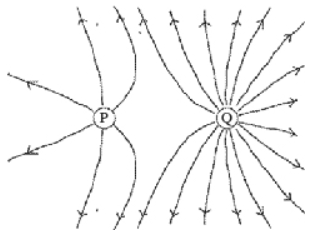The figure shows electric field lines arising from two small charged particles P and Q. Consider the following two statements:
(i) The charge on P is smaller than the charge on Q.
(ii) The electrostatic force on P is smaller than the force on Q.
Which of the above statements are true?

Both (i) and (ii) are true.

Only (i) is true.

Neither (i) nor (ii) is true.

Only (ii) is true.

Why:

3

Two point charges, Q 1 and Q 2, are separated by a distance R. If the magnitudes of both charges are doubled and their separation is also doubled, what happens to the electrical force that each charge exerts on the other one?

It remains the same.

It is reduced by a factor of 2

It increases by a factor of 4. It increases by a factor of 2.

It increases by a factor of 2

You got it correct both times :)

Why:

1)F=k Q1 Q2/ r^2

2)F=k 2Q1 2Q2/ 2r^2 = (2)(2)/ (2)^2

3)F (2)(2)/ (2)^2 =k 2Q1 2Q2/ 2r^2

4) F (4/4) = (4/4)

5) F(1) = (1)

6) F stays the same :)

4

As an electron moves in the direction the electric field lines .....

it is moving from low potential to high potential and gaining electric potential energy.

it is moving from high potential to low potential and losing electric potential energy.

it is moving from high potential to low potential and gaining electric potential energy.

it is moving from low potential to high potential and losing electric potential energy.

both its electric potential and electric potential energy remain constant.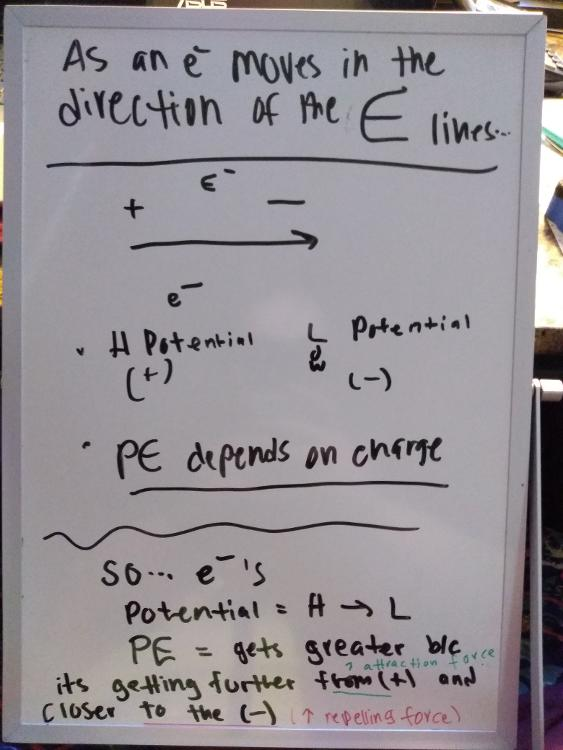You got it correct both times :)

Answer: it is moving from high potential to low potential and gaining electric potential energy.

Why: View Image :)

5

Which of the following will increase the capacitance of a parallel-plate capacitor?

an increase in the plate area and a decrease in the plate separation

an increase in the charge on the plates

an increase in the potential difference between the plates

a decrease in the plate area and an increase in the plate separation

a decrease in the potential difference between the plates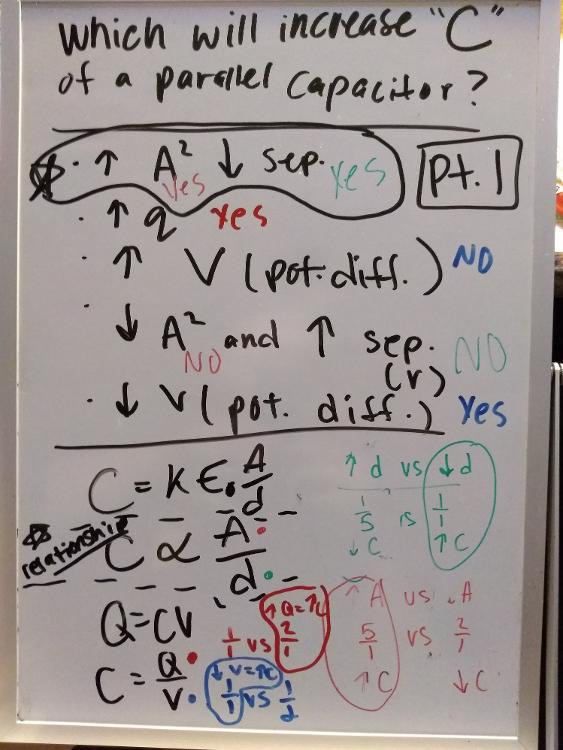You got it correct both times :)

Answer: an increase in the plate area and a decrease in the plate separation

Why: C relative A/d

View image

6

When a dielectric material is introduced between the plates of a parallel-plate capacitor and completely fills the space, the capacitance increases by a factor of 4. What is the dielectric constant of the material that was introduced?

4

None of the other choices is correct.

2

1/4

0.4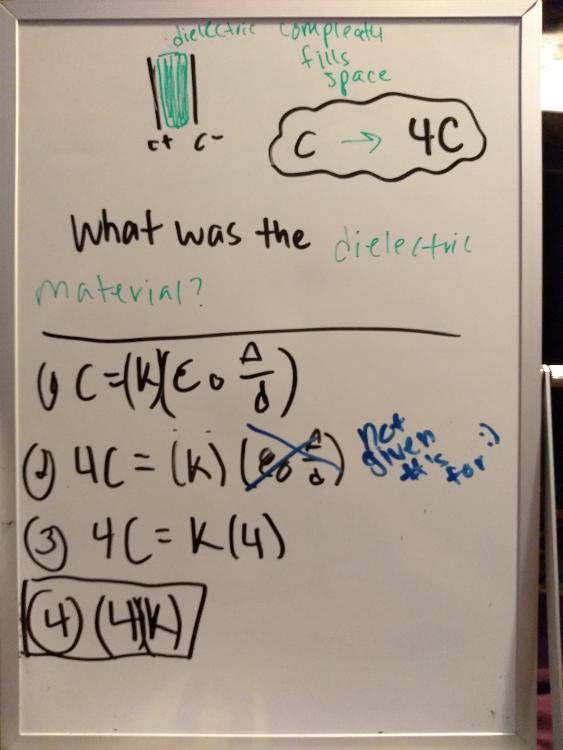It took you a minute but you got it again :)

Why: Look at the image

7

A plastic rod is charged up by rubbing a wool cloth, and brought to an initially neutral metallic sphere that is insulated from ground. It is allowed to touch the sphere for a few seconds, and then is separated from the sphere by a small distance. After the rod is separated, the rod

is attracted to the sphere.

is repelled by the sphere.

feels no force due to the sphere.

Answer: I believe it is "feels no force due to the sphere."

Why:

8

An ideal parallel-plate capacitor has a capacitance of C. If the area of the plates is doubled and the distance between the plates is halved, what is the new capacitance?

C/4

C/2

2C

4C

Why:

9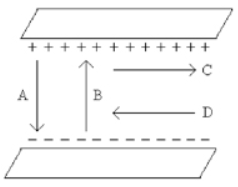Which one of the arrows shown in the figure best represents the direction of the electric field between the two uniformly charged metal plates?

A

C

B

D

None of these

You got it correct both times :)

Why: Charge is always + ----> - (somthing to fill nothing)

10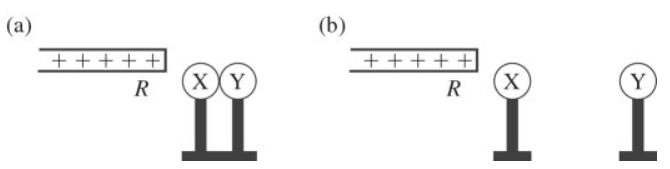X and Y are two initially uncharged metal spheres on insulating stands, and they are in contact with each other. A positively charged rod R is brought close to X as shown in part (a) of the figure. Sphere Y is now moved away from X, as shown in part (b). What are the final charge states of X and Y?

Both X and Y are negative.

X is neutral and Y is positive.

X is negative and Y is positive.

Both X and Y are neutral.

X is positive and Y is neutral.

Not Answer: Both X and Y neural

Why:

11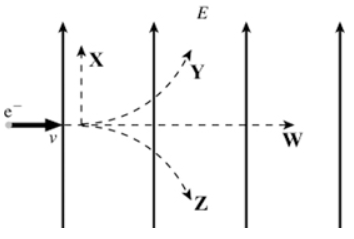An electron is initially moving to the right when it enters a uniform electric field directed upwards, as shown in the figure. Which trajectory (X, Y, Z, or W) will the electron follow in the field?

trajectory Z

trajectory X

trajectory W

trajectory Y

Answer: I believe it is "trajectory Z"

Why:

12

Two tiny beads are 25 cm apart with no other charges or fields present. Bead A carries 10 µC of charge and bead B carries 1 µC. Which one of the following statements is true about the magnitudes of the electric forces on these beads?

The force on B is 100 times the force on A.

The force on B is 10 times the force on A.

The force on A is exactly equal to the force on B.

The force on A is 100 times the force on B.

The force on A is 10 times the force on B.

Answer: I believe it is "The force on A is exactly equal to the force on B."

Why:

13

Two point charges, Q 1 and Q 2, are separated by a distance R. If the magnitudes of both charges are halved and their separation is also halved, what happens to the electrical force that each charge exerts on the other one?

It increases by a factor of 16.

It increases by a factor of 8.

It increases by a factor of 4.

It increases by a factor of 2.

It remains the same.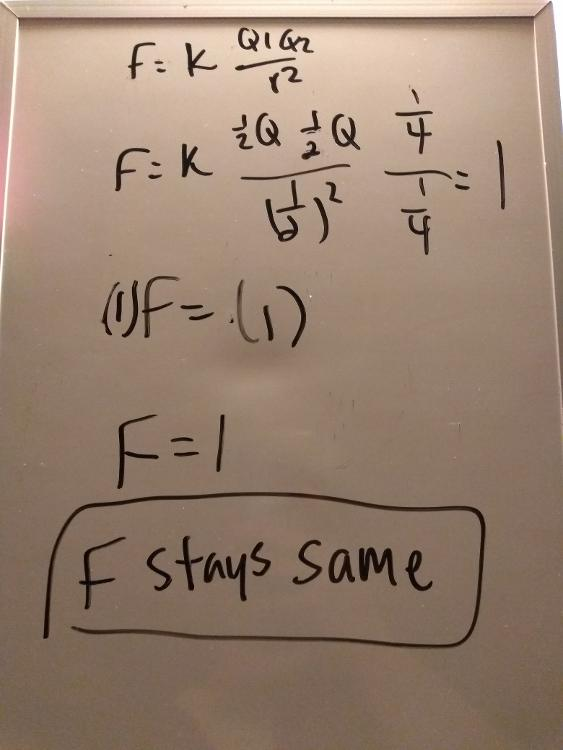You got it correct both times :)

Why: View Image

14

The electric field at point P due to a point charge Q a distance R away from P has magnitude E. In order to double the magnitude of the field at P, you could..... (Note: This was the nonsense question she removed)

reduce the distance

double the charge

reduce the distance

double the charge and at the same time reduce the distance

double the distance

Answer: I believe reduce the distance by 1/2 or double the charge

Why:

15

The electron-volt is a unit of....

electric field.

energy.

charge.

electric force.

electric potential.

Why: Because its the answer :)

16

The electric potential at a distance of 4 m from a certain point charge is 200 V relative to infinity. What is the potential (relative to infinity) at a distance of 2 m from the same charge?

400 V

600 V

200 V

100 V

50 V

Why:

17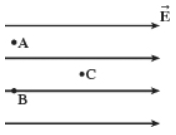A region of space contains a uniform electric field, directed toward the right, as shown in the figure. Which statement about this situation is correct?

The potentials at points A and B are equal, and the potential at point C is higher than the potential at point A.

The potential at all three locations is the same.

The potential at points A and B are equal, and the potential at point C is lower than the potential at point A.

The potential at point A is the highest, the potential at point B is the second highest, and the potential at point C is the lowest.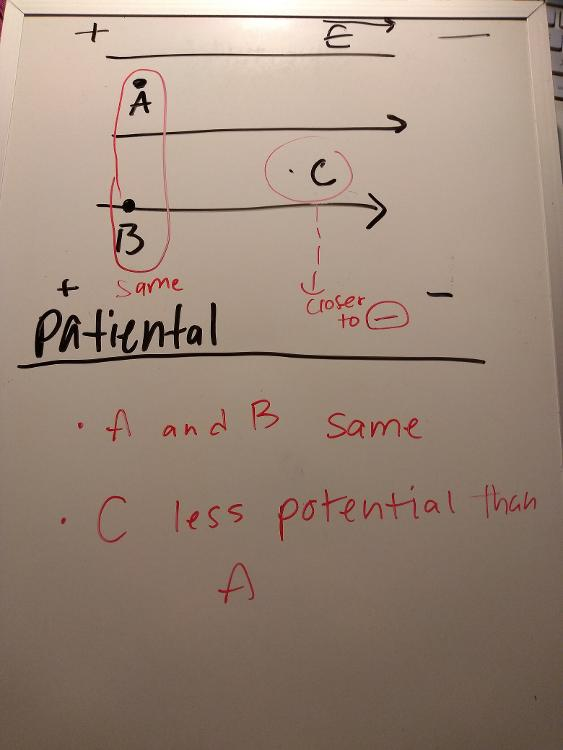You got it correct both times :)

Answer: The potential at points A and B are equal, and the potential at point C is lower than the potential at point A.

Why: View image

18

As a proton moves in a direction perpendicular to the electric field lines....

it is moving from low potential to high potential and gaining electric potential energy.

it is moving from low potential to high potential and losing electric potential energy.

it is moving from high potential to low potential and gaining electric potential energy.

both its electric potential and electric potential energy remain constant.

it is moving from high potential to low potential and losing electric potential energy.

Why:

19

A negatively-charged plastic rod is brought close to (but does not touch) a neutral metal sphere that is connected to ground. After waiting a few seconds, the ground connection is removed (without touching the sphere), and after that the rod is also removed. The sphere is now...

neutral.

negatively charged.

positively charged.

Why:

20

A capacitor consists of two large parallel plates of area A separated by a very small distance d. This capacitor is connected to a battery and charged until its plates carry charges +Q and -Q, and then disconnected from the battery. If the separation between the plates is now doubled, the potential difference between the plates will.....

be cut in half.

double.